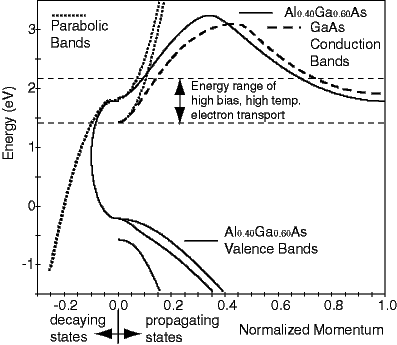# NEMO

## Realistic Dispersion Relationships

A dispersion relation has a one-to-one correspondence to the differential equation that determines the binding, propagation and attenuation of the states of the system. For a wave equation the dispersion relates the energy, E, of the plane wave, to the propagation constant, k, (psi=A*exp(-i*k*x)) and the decay constant K, (psi=A*exp(-K*x)).

Simple effective mass based approaches assume a parabolic relation (E=C*k^2) that is isotropic. However a semiconductor crystal is anisotropic (non-uniform in different directions). Moreover the symmetry of the underlying atomic wavefunctions and their coupling to the neighboring atoms resilts in a bandstructure that is non-parabolic and non-isotropic. The assumption of parabolic bands is only valid for the very bottom of the conduction band at the so-called Gamma point (Brillouine zone center).

In a GaAs/AlGaAs device various parts of the GaAs as well as the AlGaAs bandstructure are important. Electrons are free to move in the GaAs device regions and therefore we show the conduction band of the GaAs as a function of propagating electron momentum in the 100 direction (dashed line). Note that the conduction band devites from the parabolic band (dotted line) already for a a small electron mementum. This implies that the single band model predict resonance energies at energies that are too high.The AlGaAs serves as a barrier material. The conduction band edge as well as the valence band edges are shown in the solid line. To compute the tunneling probablity through a AlGaAs barrier the decay constant under the AlGaAs band edge needs to be considered. The parabolic band assumption extends a parabola from the conduction band edge to negative infinity. However the conduction and valence bands are conne3cted in the complex plane as indicated by the solic arc. The parabolic attenuation assumption overestimates the decay constant in the barriers significantly (remember there is an exponential dependence on K). With realistic bandmodels the barriers are seen to be much more transparent than the single band models would predict.

The dashed horizontal lines indicate the typical energy range of electron injection into a GaAs/AlGaAs RTD at high (room) temperatures and high applied voltages. (We found that carriers are injected to about typically 10-15 kT above the Fermi sea and result in significant current contributions.) The top dashed line indicates that there is another conduction abdn minimum in that energy range in GaAs as well as AlGaAs. Bound quantum states can form at the so-called X-point and elastic as well as ineastic tunneling through these states can occur.